# Problems of the Week

Contribute a problem

# 2018-04-02 Intermediate

An economical car consumes 4.5 kilograms of gasoline $(\ce{C8H18})$ over a distance of 100 kilometers. How much carbon dioxide $(\ce{CO2})$ is emitted over this distance?

Note: Assume that the fuel is completely burned into carbon dioxide and water vapor.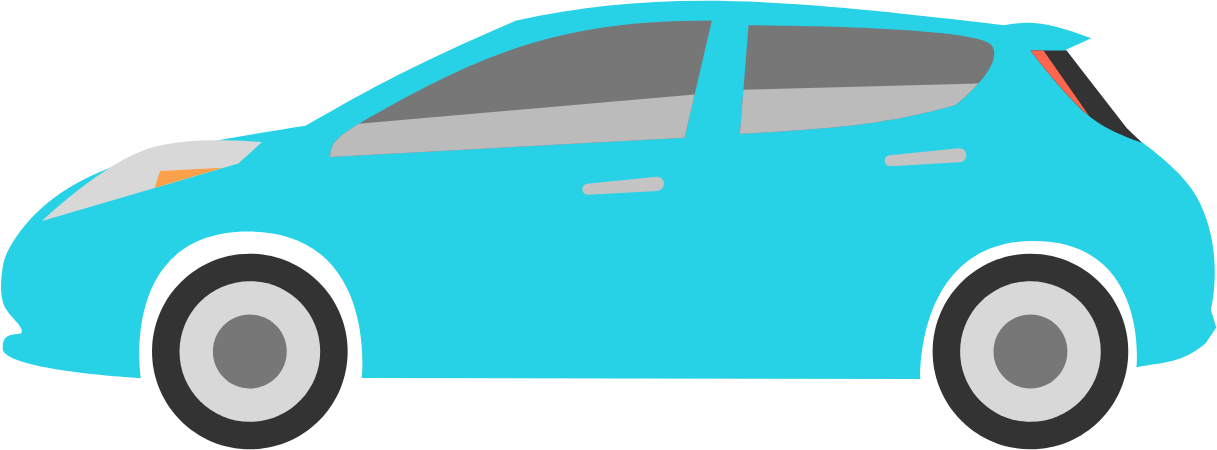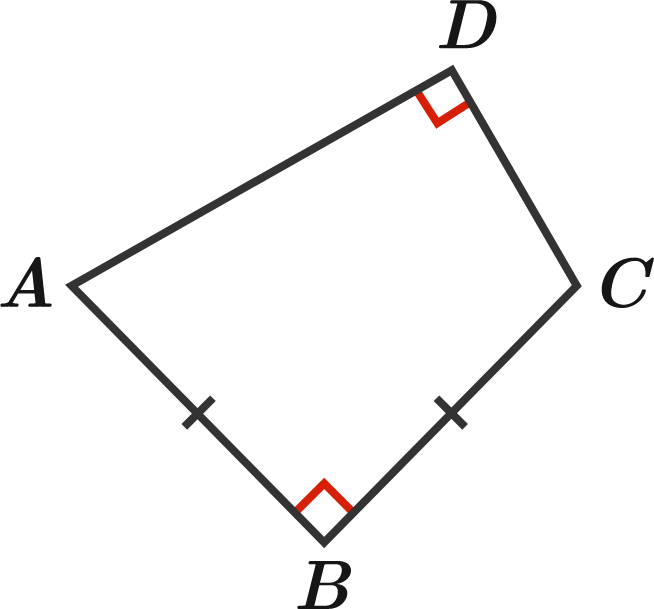Quadrilateral $ABCD$ has the following properties:

• Opposite angles $B$ and $D$ are each $90^\circ.$
• $AB$ and $BC$ have the same length.
• $AD+DC=1.$

What can be stated about other quadrilaterals that have the same properties as $ABCD?$

You select a set of $N$ specific points in the 2D plane with integer coordinates such that, for every pair of the $N$ points, the midpoint of the segment connecting them does not have integer coordinates.

What is the greatest possible value of $N?$

Below is an invalid selection of 3 points (in red), since the midpoint of $BC$ has integer coordinates.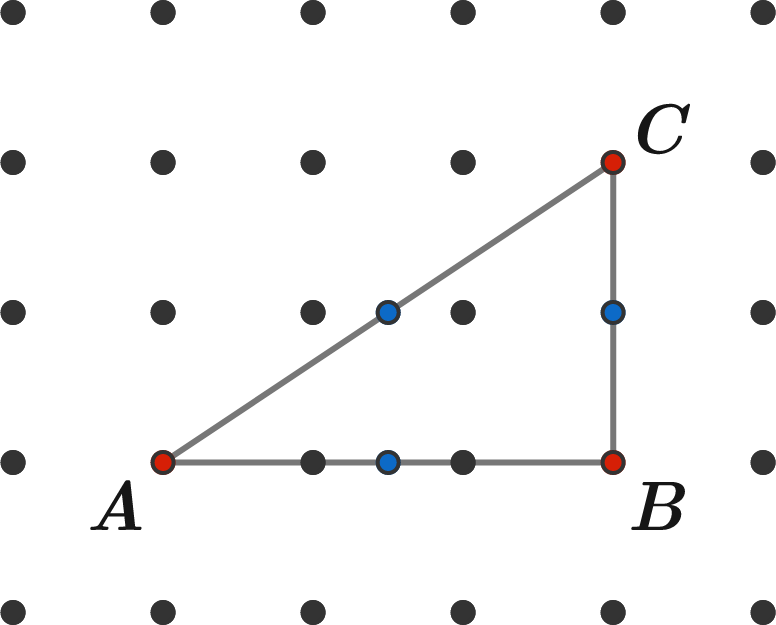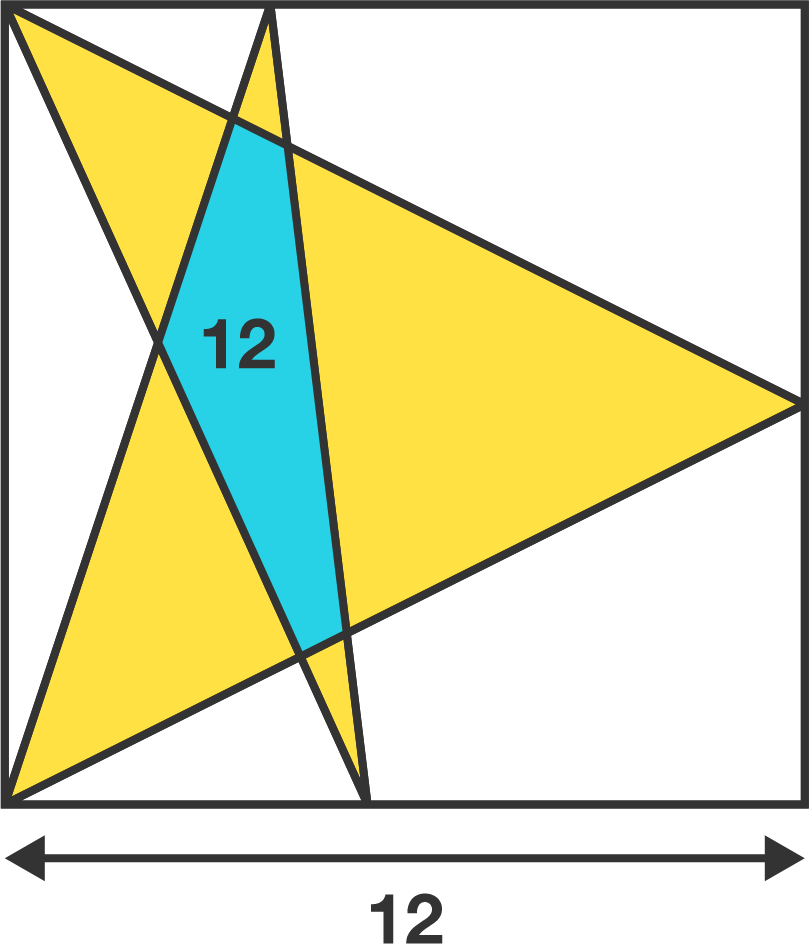The square has side length $12,$ and the area of the blue region is $12.$

What is combined area of the yellow regions?

The hero (lower right) and devil (upper left) each take turns moving on the grid with the hero going first. Both move as a King piece would on a chessboard. The devil wins if he can move to the same space as the hero. The hero wins if he can avoid the devil forever.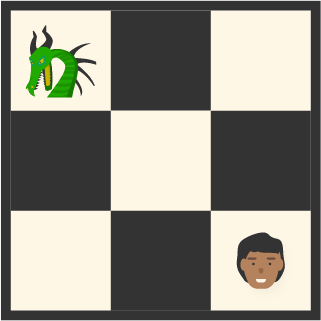The devil can always win on a 3 x 3 board.

Who will win on an 8 x 8 board?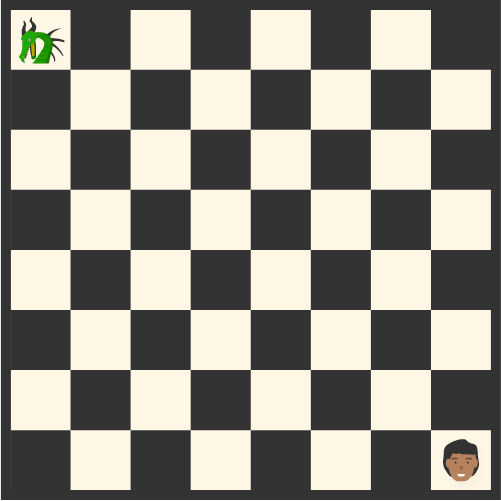×

Problem Loading...

Note Loading...

Set Loading...